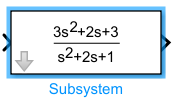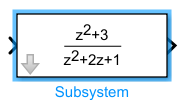# dpoly

Display transfer function on masked subsystem icon

## Syntax

``dpoly(num,den)``
``dpoly(num,den,character)``

## Description

example

````dpoly(num,den)` displays the transfer function with `num` as numerator and `den` as denominator.`dpoly(num,den,character)` displays the transfer function with the specified variable. The default character is `S`. When Simulink® draws the block icon, the initialization commands execute and the resulting equation appears on the block icon.```

## Examples

collapse all

Display a continuous transfer function in descending powers of s

`dpoly([3 2 3], [1 2 1]);`Display a discrete transfer function in descending powers of z

`dpoly([1 0 3], [1 2 1], 'z')`Display a discrete transfer function in ascending powers of 1/z

`dpoly([1 0 3], [1 2 1], 'z-')`## Input Arguments

collapse all

Numerator of the transfer function, specified as cell array.

Example: `num = [0 0 1]`

Data Types: `cell`

Denominator of the transfer function, specified as cell array.

Example: `den = [0 0 1]`

Data Types: `cell`

Variable for the transfer function, specified as a character.

Example: `dpoly(num, den, 'z')`

Data Types: `character vector` | `string`

## Version History

Introduced in R2007a